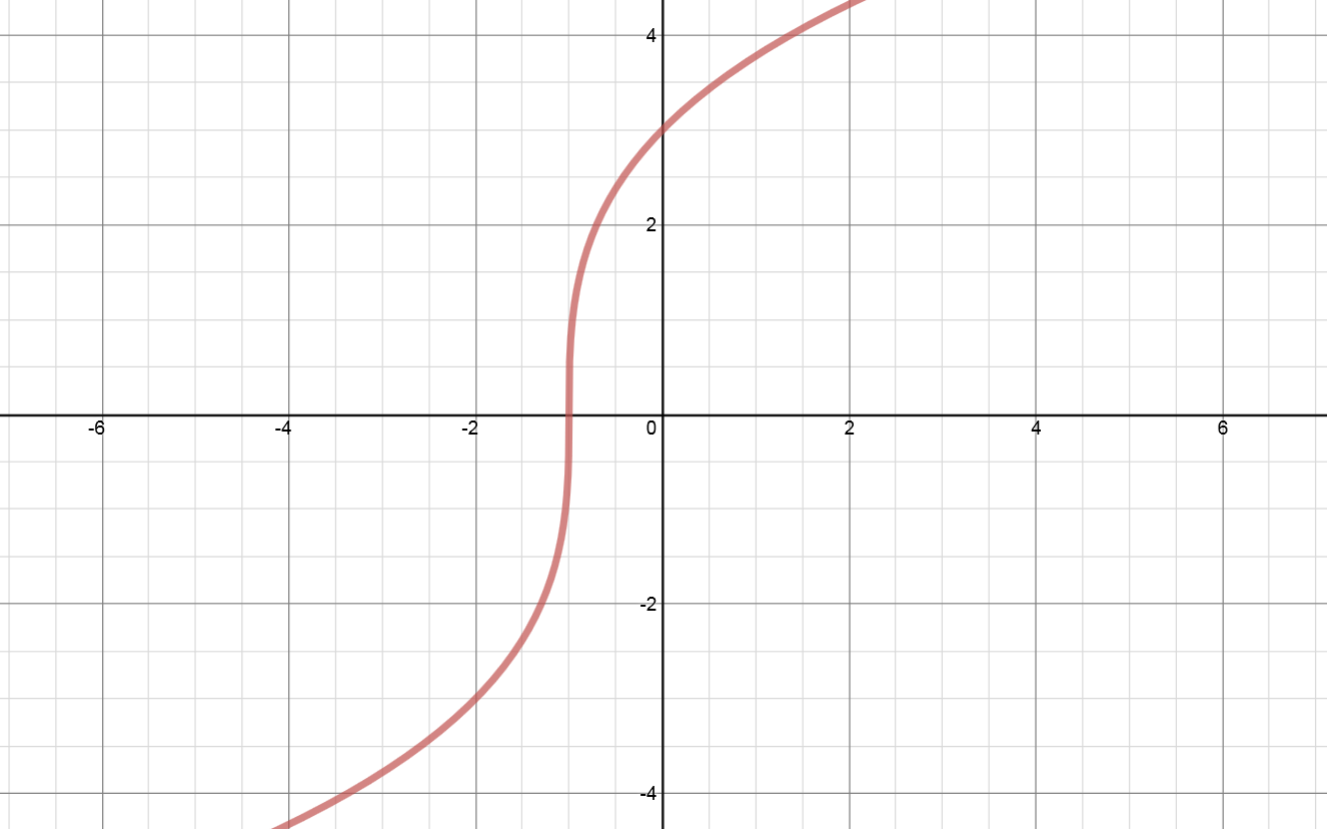# Transformations of functions: Horizontal stretches

### Transformations of functions: Horizontal stretches

#### Lessons

• Introduction
An Experiment to Study "Horizontal Stretches"
Sketch and compare: $y = {\left( {x - 4} \right)^2}$
VS.
$y = {\left( {2x - 4} \right)^2}$
VS.
$y = {\left( {\frac{x}{3} - 4} \right)^2}$
a)
Sketch all three quadratic functions on the same set of coordinate axes.

b)
Compared to the graph of $y = {\left( {x - 4} \right)^2}$:
$y = {\left( {2x - 4} \right)^2}$ is a horizontal stretch about the y-axis by a factor of _____________.
$y = {\left( {\frac{x}{3} - 4} \right)^2}$ is a horizontal stretch about the y-axis by a factor of _____________.

• 1.
Horizontal Stretches
Given the graph of $y = f\left( x \right)$ as shown, sketch:
a)
$y = f\left( {2x} \right)$

b)
$y = f\left( {\frac{1}{3}x} \right)$

c)
In conclusion:
$\left( x \right) \to \left( {2x} \right)$: horizontal stretch by a factor of ________ ⇒ all $x$ coordinates ______________________.
$\left( x \right) \to \left( {\frac{1}{3}x} \right)$: horizontal stretch by a factor of ________ ⇒ all $x$ coordinates ______________________.